Courses

# Boiling, Condensation & Heat Exchangers - MCQ Test 2

## 30 Questions MCQ Test GATE Mechanical (ME) 2022 Mock Test Series | Boiling, Condensation & Heat Exchangers - MCQ Test 2

Description
This mock test of Boiling, Condensation & Heat Exchangers - MCQ Test 2 for Mechanical Engineering helps you for every Mechanical Engineering entrance exam. This contains 30 Multiple Choice Questions for Mechanical Engineering Boiling, Condensation & Heat Exchangers - MCQ Test 2 (mcq) to study with solutions a complete question bank. The solved questions answers in this Boiling, Condensation & Heat Exchangers - MCQ Test 2 quiz give you a good mix of easy questions and tough questions. Mechanical Engineering students definitely take this Boiling, Condensation & Heat Exchangers - MCQ Test 2 exercise for a better result in the exam. You can find other Boiling, Condensation & Heat Exchangers - MCQ Test 2 extra questions, long questions & short questions for Mechanical Engineering on EduRev as well by searching above.
QUESTION: 1

### When all the conditions are identical, in the case of flow through pipeswith heat transfer, the velocity profiles will be identical for:

Solution:

Ans. (a) The velocity profile for flow through pipes with heat transfer is identical
for liquid heating and liquid cooling.

QUESTION: 2

Solution:

Ans. (b)

QUESTION: 3

### Consider the following statements:  1. If a condensing liquid does not wet a surface drop wise, then condensation will take place on it. 2. Drop wise condensation gives a higher heat transfer rate than film wise condensation. 3. Reynolds number of condensing liquid is based on its mass flowrate. 4. Suitable coating or vapour additive is used to promote film-wise condensation.Of these statements:

Solution:

Ans. (d) 1. If a condensing liquid does not wet a surface drop wise, then drop-wise
condensation will take place on it.
4. Suitable coating or vapour additive is used to promote drop-wise
condensation.

QUESTION: 4

Assertion (A): Drop-wise condensation is associated with higher heat transfer rate as compared to the heat transfer rate in film condensation.
Reason (R): In drop condensation there is free surface through which direct heat transfer takes place.

Solution:

Ans. (a)

QUESTION: 5

Consider the following statements:
The effect of fouling in a water-cooled steam condenser is that it
1. Reduces the heat transfer coefficient of water.
2. Reduces the overall heat transfer coefficient.
3. Reduces the area available for heat transfer.
4. Increases the pressure drop of waterOf these statements:

Solution:

Ans. (b) The pipe surface gets coated with deposited impurities and scale gets
formed due the chemical reaction between pipe material and the fluids. This
coating has very low thermal conductivity and hence results in high thermal
resistance. Pressure will be affected.

QUESTION: 6

Air can be best heated by steam in a heat exchanger of

Solution:

Ans. (c)

QUESTION: 7

For a balanced counter-flow heat exchanger, the temperature profilesof the two fluids are:

Solution:

Ans. (a)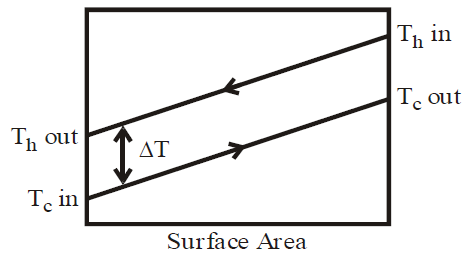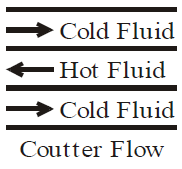QUESTION: 8

The temperature distribution curve for a heat exchanger asshown in the figure above(with usual notations) refersto which one of the following?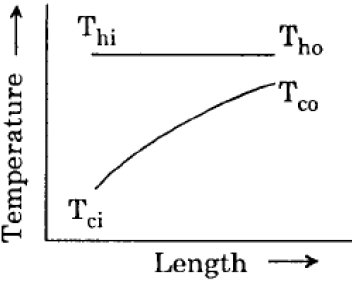Solution:

Ans. (d)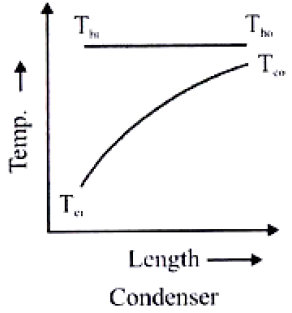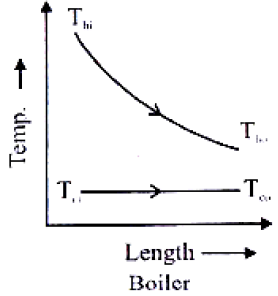QUESTION: 9

Assertion (A): Thermal conductance of heat pipe is several hundred times that of the best available metal conductor under identical conditions.
Reason (R): The value of latent heat is far greater than that of specificheat.

Solution:

Ans. (a)

QUESTION: 10

ε -NTU method is particularly useful in thermal design of heat exchangers when

Solution:

Ans. (a)

QUESTION: 11

In a counter flow heat exchanger, the product of specific heat and mass flow rate is same for the hot and cold fluids. If NTU is equal to 0.5, thenthe effectiveness of the heat exchanger is:

Solution:

Ans. (c)

QUESTION: 12

Heat pipe is widely used now-a-days because

Solution:

Ans. (c) Heat pipe can be used in different ways. Insulated portion may be made of flexible tubing to permit accommodation of different physical constraints. It can also be applied to micro-electronic circuits to maintain constant temperature. It consists of a closed pipe lined with a wicking material and containing a condensable gas. The centre portion of pipe is insulated and its two non-insulated ends respectively serve as evaporators and condensers.
Heat pipe is device used to obtain very high rates of heat flow. In practice, the thermal conductance of heat pipe may be several hundred (500) times then that
best available metal conductor, hence they act as super conductor.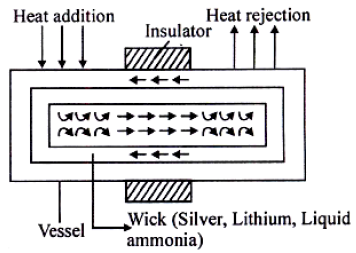QUESTION: 13

A counter flow shell - and - tube exchanger is used to heat water with hot exhaust gases. The water (Cp = 4180 J/kg°C) flows at a rate of 2 kg/swhile the exhaust gas (1030 J/kg°C) flows at the rate of 5.25 kg/s. If the heat transfer surface area is 32.5 m2 and the overall heat transfer coefficient is 200 W/m2°C, what is the NTU for the heat exchanger?

Solution:

Ans. (a)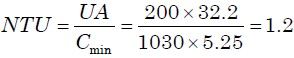QUESTION: 14

A heat exchanger with heat transfer surface area A and overall heat transfer co-efficient U handles two fluids of heat capacities Cmax and Cmin. The parameter NTU (number of transfer units) used in the analysis of heat exchanger is specified as

Solution:

Ans. (d)

QUESTION: 15

A cross-flow type air-heater has an area of 50 m2. The overall heattransfer coefficient is 100 W/m2K and heat capacity of both hot and coldstream is 1000 W/K. The value of NTU is:

Solution:

Ans. (c)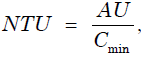A = Area = 50m2

U = Overall heat transfer coefficient 100W/m2 K

Cmin = Heat capacity = 1000W/K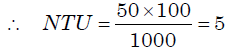QUESTION: 16

The equation of effectiveness ε = 1 − e−NTU of a heat exchanger is valid(NTU is number or transfer units) in the case of:

Solution:

Ans. (c)

QUESTION: 17

In a balanced counter flow heat exchanger with ,  Mh Ch = McCc, the NTUis equal to 1.0. What is the effectiveness of the heat exchanger?

Solution:

Ans. (a) In this case the effectiveness of the heat exchanger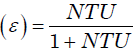QUESTION: 18

A heat exchanger with heat transfer surface area A and overall heattransfer coefficient U handles two fluids of heat capacities C1, and C2,such that C1 > C2. The NTU of the heat exchanger is given by:

Solution:

Ans. (a) NTU (number of transfer units) used in analysis of heat exchanger is
specified as AU/Cmin.

QUESTION: 19

After expansion from a gas turbine, the hot exhaust gases are used toheat the compressed air from a compressor with the help of a crossflow compact heat exchanger of 0.8 effectiveness. What is the numberof transfer units of the heat exchanger?

Solution:

Ans. (b) Effectiveness,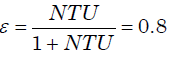QUESTION: 20

When tc1 and tc2 are the temperatures of cold fluid at entry and exit respectively and th1 and th2 are the temperatures of hot fluid at entry and exit point, and cold fluid has lower heat capacity rate as compared
to hot fluid, then effectiveness of the heat exchanger is given by:

Solution:

Ans. (d)

QUESTION: 21

A designer chooses the values of fluid flow ranges and specific heats insuch a manner that the heat capacities of the two fluids are equal. Ahot fluid enters the counter flow heat exchanger at 100°C and leaves at60°C. The cold fluid enters the heat exchanger at 40°C. The mean temperature difference between the two fluids is:

Solution:

Ans. (d) Mean temperature difference
= Temperature of hot fluid at exit – Temperature of cold fluid at entry
= 60° – 40° = 20°C

QUESTION: 22

For evaporators and condensers, for the given conditions, the logarithmic mean temperature difference (LMTD) for parallel flow is:

Solution:

Ans. (a)

QUESTION: 23

Assertion (A): The LMTD for counter flow is larger than that of parallelflow for a given temperature of inlet and outlet.
Reason (R): The definition of LMTD is the same for both counter flowand parallel flow.

Solution:

Ans. (b) Both statements are correct but R is not exactly correct explanation for A.

QUESTION: 24

Assertion (A): It is not possible to determine LMTD in a counter flow heat exchanger with equal heat capacity rates of hot and cold fluids.

Reason (R): Because the temperature difference is invariant along the length of the heat exchanger.

Solution:

Ans. (d)

QUESTION: 25

Match List-I with List-II and select the correct answer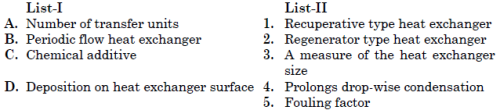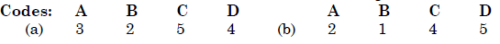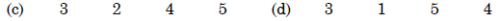Solution:

Ans. (c)

QUESTION: 26

In a heat exchanger, the hot liquid enters with a temperature of 180ºCand leaves at 160ºC. The cooling fluid enters at 30ºC and leaves at110ºC. The capacity ratio of the heat exchanger is: [IES-2010]

Solution:

Ans. (a) Capacity ratio of heat exchanger =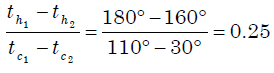QUESTION: 27

Which one of the following diagrams correctly shows the temperature distribution for a gas-to-gas counterflow heat exchanger?

Solution:

Ans. (b)

QUESTION: 28

Assertion (A): A counter flow heat exchanger is thermodynamically more efficient than the parallel flow type.
Reason (R): A counter flow heat exchanger has a lower LMTD for the same temperature conditions.

Solution:

Ans. (c)

QUESTION: 29

Consider the following statements:
In a shell and tube heat exchanger, baffles are provided on the shellside to:
1. Prevent the stagnation of shell side fluid
2. Improve heat transfer
3. Provide support for tubes
Select the correct answer using the codes given below:

Solution:

Ans. (d) Baffles help in improving heat transfer and also provide support for
tubes.

QUESTION: 30

Match List-I (Application) with List-II (Type of heat exchanger) and select the correct answer using the code given below the lists:
List-I                                                                            List-II
A. Gas to liquid                                                         1. Compact
B. Space vehicle                                                       2. Shell and Tube
C. Condenser                                                            3. Finned tube
D. Air pre-heater                                                       4. Regenerative
Codes: A B C D                                    A B C D
(a) 2 4 3 1                                 (b) 3 1 2 4
(c) 2 1 3 4                                 (d) 3 4 2 1

Solution:

Ans. (b)